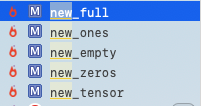Bonky Zhu
If someone is able to show me that what I think or do is not right, I will happily change, for I seek the truth, by which no one was ever truly harmed. It is the person who continues in his self-deception and ignorance who is harmed.

Pytorch 基础

Pytorch基于Python的科学计算包，服务于以下两种场景:

• 作为NumPy的替代品，可以使用GPU的强大计算能力
• 提供最大的灵活性和高速的深度学习研究平台

基本

Tensor

>>> x = torch.empty(5, 3) >>> x tensor([[0.0000e+00, 2.0000e+00, 0.0000e+00],         [2.0000e+00, 2.7648e-35, 1.4013e-45],         [2.7674e-35, 1.4013e-45, 2.7619e-35],         [1.4013e-45, 2.7675e-35, 1.4013e-45],         [2.7620e-35, 7.9463e+08, 5.0944e-14]])

x = torch.rand(5, 3)

x = torch.zeros(5, 3, dtype=torch.long)

>>> x = torch.tensor([5.5, 3]) >>> print(x)

x = x.new_ones(5, 3, dtype=torch.double)      # new_* 方法来创建对象 x = torch.randn_like(x, dtype=torch.float)    # 覆盖 dtype! 对象的size 是相同的，只是值和类型发生了变化运算

加减法，转置

Pytorch一共提供了四种加法，同样地减法也有函数 sub(不会改变 x)，sub_(会改变 x)。转置是 tt_

x = torch.rand(5, 3) y = torch.rand(5, 3) #第一种 print(x + y) #第二种 print(torch.add(x, y)) #第三种 result = torch.empty(5, 3) torch.add(x, y, out=result) print(result) #第四种，注意这里原变量 y 会改变 y.add_(x) print(y)

x.mul(y)

矩阵相乘 mm()

x.mm(y)

x 和 y 需要满足矩阵乘法的维度关系

数乘 *

Tensor与标量k*乘法的结果是Tensor的每个元素乘以k

>>> a = torch.ones(3,4) >>> a tensor([[1., 1., 1., 1.],         [1., 1., 1., 1.],         [1., 1., 1., 1.]]) >>> a * 2 tensor([[2., 2., 2., 2.],         [2., 2., 2., 2.],         [2., 2., 2., 2.]])

>>> a = torch.ones(3,4) >>> a tensor([[1., 1., 1., 1.],         [1., 1., 1., 1.],         [1., 1., 1., 1.]]) >>> b = torch.Tensor([1,2,3]).reshape((3,1)) >>> b tensor([[1.],         [2.],         [3.]]) >>> a * b tensor([[1., 1., 1., 1.],         [2., 2., 2., 2.],         [3., 3., 3., 3.]])

• 如果 y=m那么，a * b = a.mm(b)
• 如果 x=m 或者 y=n或者 a,b 有一个为常数, 那么a * b = a.mul(b)
• 否则都是不合法的运算

其他运算

• 索引或切片运算，与NumPy索引方式相同
• torch.view: 可以改变张量的维度和大小，与Numpyreshape相同
• 如果你有只有一个元素的张量，使用.item()来得到Python数据类型的数值（返回的是一个常数，可以 Tensor 转常数）
• Numpy 的互相转换：
• TensorNumpy - 使用 a.numpy()
• 注意这里返回的只是个引用，b 始终等于 a （我感觉说不定和计算图也有点关系）：
• >>> a = torch.ones(5)
• >>> b = a.numpy()